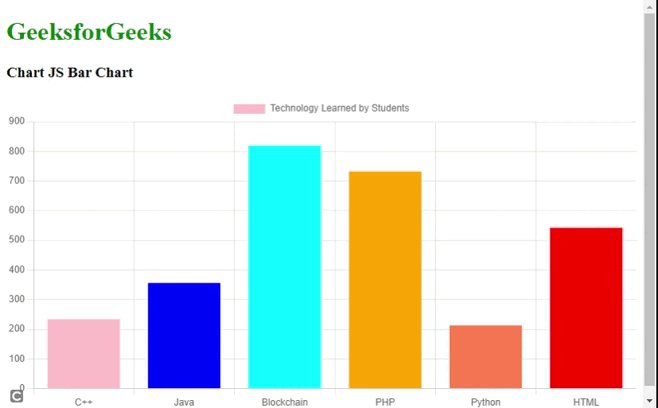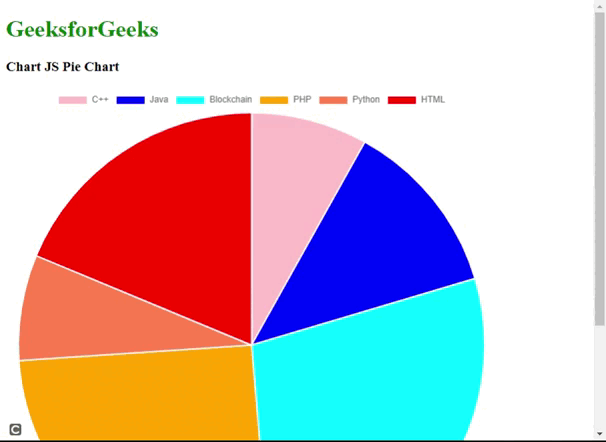Open in App
Not now

# How to implement bar and pie charts using Chart.js ?

• Last Updated : 24 Jan, 2023

In this article, we will learn to implement basic bar graphs and pie charts using the Chart JS CDN library.

Approach:

1. In the HTML design, use the <canvas> tag for showing the bar or pie chart graph.
2. In the script part of the code, instantiate the ChartJS object by setting the type, data and options properties of the library.
• type: The type can take values like “pie”,”bar”, and “line” by the ChartJS library.
• data: It sets the labels and datasets that contain the data array and other display-related properties.
• options: It sets the axis direction, title chart, and display flag as a boolean value as true or false.

<script src=”https://cdnjs.cloudflare.com/ajax/libs/Chart.js/4.1.2/chart.umd.js”></script>

Example 1: The following code shows a basic vertical graph for the students learning various computer science technology using the Chart JS library.

## HTML

 ` ` `<``html``> ` `<``head``> ` `    ``<``title``>Chart JS Bar Chart ` `    ``<``script` `src``= ` `"https://ajax.googleapis.com/ajax/libs/jquery/3.6.1/jquery.min.js"``> ` `    `` ` `    ``<``script` `src``= ` `"https://cdnjs.cloudflare.com/ajax/libs/Chart.js/4.1.2/chart.umd.js"``> ` `    `` ` ` ` `<``body``> ` `    ``<``div``> ` `        ``<``h1` `style``=``"color:green"``>GeeksforGeeks ` `        ``<``h3``>Chart JS Bar Chart ` `        ``<``div``> ` `            ``<``canvas` `id``=``"barChartID"``> ` `        `` ` `    `` ` `    `  `    ``<``script``> ` `        ``// Bar chart ` `        ``new Chart(\$("#barChartID"), { ` `            ``type: 'bar', ` `            ``options: { ` `                ``legend: { display: true }, ` `                ``indexAxis: 'x', ` `                ``title: { ` `                    ``display: true, ` `                    ``text: 'Bar Chart using ChartJS library' ` `                ``} ` `            ``}, ` `            ``data: { ` `                ``labels: ["C++", "Java", "Blockchain", "PHP", "Python","HTML"], ` `                ``datasets: [ ` `                    ``{ ` `                        ``label: "Technology Learned by Students", ` `                        ``backgroundColor: ["#FFC0CB", "#0000FF", ` `                            ``"#00FFFF", "#FFA500", "#FF7F50","#FF0000"], ` `                        ``data: [234, 356, 819, 732, 213, 542] ` `                    ``} ` `                ``] ` `            ``}             ` `        ``}); ` `    `` ` ` ` ``

Output:Example 2: The following code shows a basic pie chart graph for the students learning various computer science technology using the Chart JS library.

## HTML

 ` ` `<``html``> ` `<``head``> ` `    ``<``title``>Chart JS Bar Chart ` `    ``<``script` `src``= ` `"https://ajax.googleapis.com/ajax/libs/jquery/3.6.1/jquery.min.js"``> ` `    `` ` `    ``<``script` `src``= ` `"https://cdnjs.cloudflare.com/ajax/libs/Chart.js/4.1.2/chart.umd.js"``> ` `    `` ` ` ` `<``body``> ` `    ``<``div``> ` `        ``<``h1` `style``=``"color:green"``>GeeksforGeeks ` `        ``<``h3``>Chart JS Bar Chart ` `        ``<``div``> ` `            ``<``canvas` `id``=``"barChartID"``> ` `        `` ` `    `` ` `    `  `    ``<``script``> ` `        ``// Bar chart ` `        ``new Chart(\$("#barChartID"), { ` `            ``type: 'bar', ` `            ``options: { ` `                ``legend: { display: true }, ` `                ``indexAxis: 'x', ` `                ``title: { ` `                    ``display: true, ` `                    ``text: 'Bar Chart using ChartJS library' ` `                ``} ` `            ``}, ` `            ``data: { ` `                ``labels: ["C++", "Java", "Blockchain", "PHP", "Python","HTML"], ` `                ``datasets: [ ` `                    ``{ ` `                        ``label: "Technology Learned by Students", ` `                        ``backgroundColor: ["#FFC0CB", "#0000FF", ` `                            ``"#00FFFF", "#FFA500", "#FF7F50","#FF0000"], ` `                        ``data: [234, 356, 819, 732, 213, 542] ` `                    ``} ` `                ``] ` `            ``}             ` `        ``}); ` `    `` ` ` ` ``

Output:My Personal Notes arrow_drop_up
Related Articles